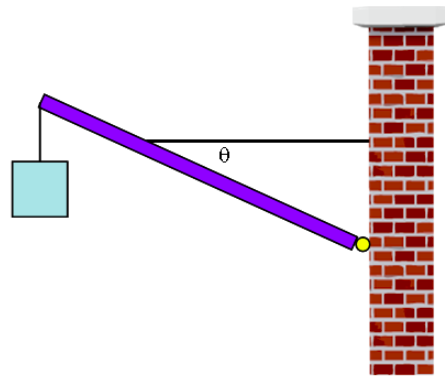# Problem: A purple beam is hinged to a wall to hold up a blue sign. The beam has a mass of mb = 6.3 kg and the sign has a mass of ms = 15.1 kg. The length of the beam is L = 2.74 m. The sign is attached to the very end of the beam, but the horizontal wire holding up the beam is attached 2/3 of the way to the end of the beam.The angle the wire makes with the beam is θ = 31.7°.1) What is the tension in the wire?2) What is the net force the hinge exerts on the beam?3) The maximum tension the wire can have without breaking is T = 804 N. What is the maximum mass sign that can be hung from the beam?4) What else could be done in order to be able to hold a heavier sign?a.) while still keeping it horizontal, attach the wire to the end of the beamb.) keeping the wire attached at the same location on the beam, make the wire perpendicular to the beamc.) attach the sign on the beam closer to the walld.) shorten the length of the wire attaching the box to the beam

###### FREE Expert Solution

In this problem, we'll be dealing with the sum of forces and torques.

Torque equations:

$\overline{){\mathbit{\tau }}{\mathbf{=}}{\mathbit{r}}{\mathbit{F}}{\mathbf{sin}}{\mathbit{\theta }}}$

Sum of forces for a system in equilibrium (Newton's second law):

$\overline{){\mathbf{\Sigma }}{\mathbf{F}}{\mathbf{=}}{\mathbf{0}}}$

Sum of torques for a system in equilibrium:

$\overline{){\mathbf{\Sigma }}{\mathbf{\tau }}{\mathbf{=}}{\mathbf{0}}}$

100% (379 ratings)###### Problem DetailsA purple beam is hinged to a wall to hold up a blue sign. The beam has a mass of mb = 6.3 kg and the sign has a mass of ms = 15.1 kg. The length of the beam is L = 2.74 m. The sign is attached to the very end of the beam, but the horizontal wire holding up the beam is attached 2/3 of the way to the end of the beam.The angle the wire makes with the beam is θ = 31.7°.

1) What is the tension in the wire?
2) What is the net force the hinge exerts on the beam?
3) The maximum tension the wire can have without breaking is T = 804 N. What is the maximum mass sign that can be hung from the beam?
4) What else could be done in order to be able to hold a heavier sign?
a.) while still keeping it horizontal, attach the wire to the end of the beam
b.) keeping the wire attached at the same location on the beam, make the wire perpendicular to the beam
c.) attach the sign on the beam closer to the wall
d.) shorten the length of the wire attaching the box to the beam